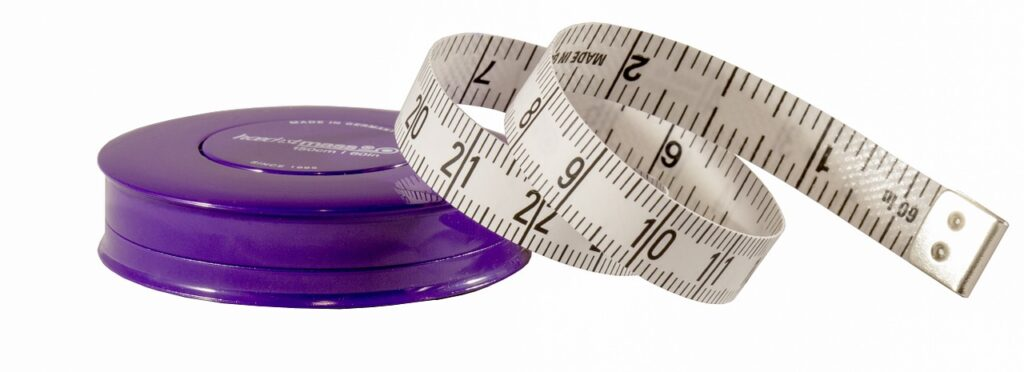# What you need to know for O Level Physics Chapter: Physical Quantities, Units and MeasurementIn O Level Physics, physical quantities, units and measurement is the first chapter students learn. In this post, let’s talk about what you need to know for this chapter. If you prefer to watch rather than read, check out our Youtube video on what’s tested in this chapter for O level physics.

In this chapter, students learn that they should include magnitude and units whenever they are measuring anything that is measurable. In Singapore, and also in the O Level syllabus, we often us metric units. Hence, students learn the SI unit for common physical quantities. We also use prefix if something is very small or big compared to the SI units. Finally, the chapter ends of with measurement of length and time. If you take a look at the syllabus, the drawing of vector diagram comes with this chapter, but most school include it in the chapter on forces, since we usually draw vector diagram involving forces in O Level Physics.

This chapter is really easy to study, and the questions from this chapter are usually found in paper 1 (multiple choice questions). By the time you are revising for O level, this topic shouldn’t take more than half an hour for revision.

## Checklist of what’s tested in Physical quantities, units and measurement (O Level Physics)

Here’s a checklist of what you need to know once you’ve completed revision of this chapter on physical quantities, units and measurement:

☐ physical quantities consist of magnitude and units

☐ know the base quantities and their units (for mass, current, length, time, temperature and amount of substance)

☐ prefixes and their symbols

☐ order of magnitude of common objects

☐ common instruments used to measure length i.e. tapes, rules, vernier calipers, micrometer

☐ how to measure short intervals of time using the stopwatch

☐ how to measure a period of a pendulum

☐ scalars and vectors and drawing of vector diagram (often combined with the chapter on Forces in O Level Physics)

We talked about this O Level chapter on physical quantities, units and measurement in detail in this video below:

error: Content is protected !!